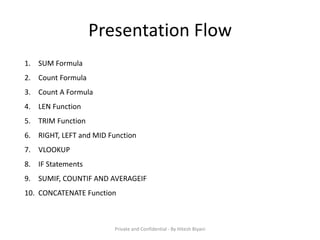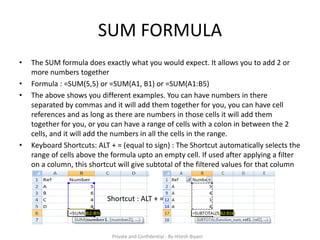Se está descargando tu SlideShare. ×

Anuncio
Anuncio
Anuncio
Anuncio
Anuncio
Anuncio
Anuncio
Anuncio
Anuncio
Anuncio
AnuncioCargando en…3
×

## Eche un vistazo a continuación

1 de 14 Anuncio

These are some basic and moderate excel formulas but are widely used in a corporate world be it any industry. A must read for freshers looking to seek a job with profiles in Banking, Insurance, BPO / KPO (Data support), etc

These are some basic and moderate excel formulas but are widely used in a corporate world be it any industry. A must read for freshers looking to seek a job with profiles in Banking, Insurance, BPO / KPO (Data support), etc

Anuncio
Anuncio

1. 1. Excel Formulas 10 Formulas that helps you in any JOB
2. 2. Presentation Flow 1. SUM Formula 2. Count Formula 3. Count A Formula 4. LEN Function 5. TRIM Function 6. RIGHT, LEFT and MID Function 7. VLOOKUP 8. IF Statements 9. SUMIF, COUNTIF AND AVERAGEIF 10. CONCATENATE Function Private and Confidential - By Hitesh Biyani
3. 3. SUM FORMULA • The SUM formula does exactly what you would expect. It allows you to add 2 or more numbers together • Formula : =SUM(5,5) or =SUM(A1, B1) or =SUM(A1:B5) • The above shows you different examples. You can have numbers in there separated by commas and it will add them together for you, you can have cell references and as long as there are numbers in those cells it will add them together for you, or you can have a range of cells with a colon in between the 2 cells, and it will add the numbers in all the cells in the range. • Keyboard Shortcuts: ALT + = (equal to sign) : The Shortcut automatically selects the range of cells above the formula upto an empty cell. If used after applying a filter on a column, this shortcut will give subtotal of the filtered values for that column Shortcut : ALT + = Private and Confidential - By Hitesh Biyani
4. 4. COUNT Formula • The count formula counts the number of cells in a range that have numbers in them • This formula only works with numbers though, It only counts the cells where there are numbers • Formula : =Count(A1:A10) Private and Confidential - By Hitesh Biyani
5. 5. COUNTA Formula • Counts the number of non-empty cells in a range. It will count cells that have numbers and/or any other characters in them • Formula: =COUNTA(A1:A10) • It counts the number of non-empty cells no matter the data type. Private and Confidential - By Hitesh Biyani
6. 6. LEN Function • The LEN formula counts the number of characters in a cell. Be careful though! This includes spaces. • Formula : =LEN(A1) • Notice the difference in the formula results: 10 characters without spaces in between the words, 12 with spaces between the words. Private and Confidential - By Hitesh Biyani
7. 7. TRIM Function • Useful for removing extra spaces in a cell whether at beginning, trailing or anywhere between the words. • Often when you pull data from a database you may find some extra spaces are put in behind or in front of legitimate data. • This can wreak havoc if you are trying to compare using IF statements or VLOOKUP’s. • Formula: =TRIM(A1) • I added in an extra space behind “I Love Excel”. The TRIM formula removes that extra space. Check out the character count difference with and without the TRIM formula. Private and Confidential - By Hitesh Biyani
8. 8. RIGHT, LEFT and MID function • These Formulas return the specified number of characters from a text string • Right gives you the number of specified characters from right of a text string • Left gives you the number of specified characters from left of a text string • MID gives you the number of specified characters from middle of a text string • Formula: =RIGHT(Text or Cell reference, number of characters), = LEFT(Text or Cell reference, number of characters) and =MID(Text or Cell reference, Start Number, number of characters) I used the LEFT formula to get the first word. I had it look in cell A1 and grab only the 1st character from the left. This gave us the word “I” from “I love Excel” I used the MID formula to get the middle word. I had it look in cell A1, start at character 3, and grab 5 characters after that. This gives us just the word “love” from “I love Excel” I used the RIGHT formula to get the last word. I had it look at cell A1 and grab the first 5 characters from the right. This gives us “Excel” from “I love Excel” Private and Confidential - By Hitesh Biyani
9. 9. VLOOKUP Function • By far the most used function in day to day operations of many businesses • What it Does : Looks for a value in another table or array and returns value in the same row from a column you specify • In Simple terms, it does a find action of a value from one sheet or table or file in another sheet or table or file and copies the value corresponding to that value from the column specified in the formula • Formula: =Vlookup(lookup_value, table_array, col_index_num, range_lookup) • Let’s Break the above formula in 4 parts – a. Lookup_Value = This is the field that has common references / values to other file which has more details b. Table_Array = This is the range that you select from another file or sheet or table starting from the column which has common references / values to the lookup_value as defined in (a) above c. Col_index_num = This is the column reference number from which you wish to pick values against the lookup value as defined in (a) above d. range_lookup = This is used to derive approximate or exact match. “0” or “False” value will provide an exact match whereas “1” or “True” value will provide an approximate match Private and Confidential - By Hitesh Biyani
10. 10. VLOOKUP Function (Contd..) • Example • In this example, I am attempting to create Master List from List 1 and List 2 using Sales ID Person as my “Lookup _value” • Some Points to remember, a. For a vlookup function, there always need to be a common field with common values which is referred to as “lookup_value”. b. It can be read like this while attempting the formula, lookup “x” in this range i.e. “x:y” and pick up “y” and do an exact match i.e. “False” or “0” Lookup_value Col_index_num Range_lookupTable_Array Private and Confidential - By Hitesh Biyani
11. 11. IF Statements • This again is most used formula for a lot of analytical scenarios where a data has to react differently based on different situations • Formula: =IF(logical_test, value_if_true, value_if_false) • Logical Statements may include comparison of one value to another using “>”, “<“, “=“, “OR”, “AND”, etc • In simple terms, it looks if the logical statement returns a true value or false value and accordingly, user can define the value to be displayed if the result is true and and value to be displayed if result is false. So basically it has 3 parts i.e. IF (logical test), THEN (if true) and ELSE (If not true) • Let’s Break the above formula in 3 parts – a. Logical Statement – Usually a comparison statement using Logical Operators b. True value – If the answer to the logical statement is true, the value specified for true value will be returned c. False Value - If the answer to the logical statement is false, the value specified for false value will be returned • Example Private and Confidential - By Hitesh Biyani
12. 12. IF Statements(Contd…) • If Statement is a very powerful formula which can be used to derive results of complex problems. It can be used to avoid multiple steps while working on a worksheet and instead can give result in one shot. This can be done using Nested IF Statements. This is covered in our Advanced Excel Program • Example of a Nested IF Statement • =if(logical_test, value_if_true, if(logical_test, value_if_true, if(logical_test, so on and so forth) • Nested IF always has a new IF Statement for False value. This argument can be continued till all the situations are covered. This statement is useful for defining Age Buckets • =if(age<7, “0-7”,if(7<age<15, “8-15”, if(15<age<30, “16-30”, if(30<age<60, “31-60”, “60 and above”)))) • There is one more version of IF Statements named IFERROR • This formula will be triggered if the other formulas wind up giving you an error. It could look something like #N/A and that’s pretty ugly. This can happen for legitimate reasons, but you don’t want to hand your boss a sheet full of #N/A symbols • In the above example to avoid #N/A values, below formula can be used • =iferror(if(age<7, “0-7”,if(7<age<15, “8-15”, if(15<age<30, “16-30”, if(30<age<60, “31-60”, “60 and above”)))),””) Private and Confidential - By Hitesh Biyani
13. 13. SUMIF, COUNTIF AND AVERAGEIF • These Formulas do their respective functions i.e. if the criteria are met • Formulas: =SUMIF(Range, Criteria, Sum_Range) | =COUNTIF(Range, Criteria) | =AVERAGEIF(Range, Criteria, Average_Range) • In Simple Terms, it provides a sum of values within cells, count of cells and average of values within cells for the criteria if satisfied • Examples Range Criteria Sum_Range Average_Range Private and Confidential - By Hitesh Biyani
14. 14. CONCATENATE • Simple yet a very effective and useful formula for many day-to-day operations in office. • This Formula is also denoted by Ampersand (&) Sign and it is used for joining the values in difference cells • Many a times, we get a database where we feel the need to combine 2 columns or 2 fields, this formula helps you achieve that in no time. • Example • In the above example, I have used Concatenate formula in Column D to derive Full Names from the information given in Columns A, B and C • Formula: = Concatenate(A2, “ ”, B2, “ ”, C2) OR =A2&“ ”&B2&“ ”&C2. • In the above formula, I have combined the First Name, Middle Name and Surname with Spaces between each of them. The SPACE or any Text has to be denoted in “Quotation” Marks to be reflected in the result Private and Confidential - By Hitesh Biyani